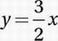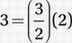# SAT Math Multiple Choice Question 617: Answer and Explanation

### Test Information

Question: 617

2. 3x+ 4y = 18Which of the following ordered pairs (x, y) is a solution of the system of equations above?

• A. (2, 3)
• B. (3, 2.25)
• C. (4, 1.5)
• D. (4, 6)

y = 3 is a solution because 3(2) + 4(3) = 18, and.